SCERT AP 7th Class Maths Solutions Pdf Chapter 9 Algebraic Expressions Ex 9.3 Textbook Exercise Questions and Answers.

## AP State Syllabus 7th Class Maths Solutions 9th Lesson Algebraic Expressions Ex 9.3

Question 1.
Write standard form and additive inverse of the following expressions.
(i) – 6a
Additive inverse of – 6a = – (- 6a) = 6a(ii) 2 + 7c2
Standard form of 2 + 7c2 = 7c2 + 2
Additive inverse of 7c2 + 2
= – (7c2 + 2)
= – 7c2 – 2

(iii) 6x2 + 4x – 5
Given expression is in standard form.
Additive inverse of 6x2 + 4x – 5
= – (6x2 + 4x – 5)
= – 6x2 – 4x + 5

(iv) 3c + 7a – 9b
Standard form of 3c + 7a – 9b = 7a – 9b + 3c
Additive inverse of 7a – 9b + 3c
= – (7a – 9b + 3c)
= – 7a + 9b – 3c

Question 2.
Write the following expressions in standard form:
(i) 6x + x2 – 5
Standard form of 6x + x2 – 5
= x2 + 6x – 5

(ii) 3 – 4a2 – 5a
Standard form of 3 – 4a2 – 5a
= – 4a2 – 5a + 3

(iii) – m + 6 + 3m2
Standard form of – m + 6 + 3m2
= 3m2 – m + 6(iv) c3 + 1 + c + 2c2
Standard form of
c3 + 1 + c + 2c2 = c3 + 2c2 + c + 1

(v) 9 – p2
Standard form of 9 – p2 = – p2 + 9

Question 3.
Add the following algebraic expressions using both horizontal and vertical methods. Did you get the same answer with both the methods? Verify.
(i) 2x2 – 6x +3; 4x2 + 9x + 5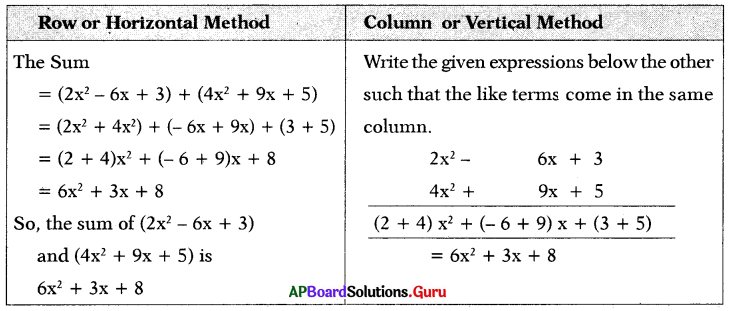(ii) a2 + 6ab + 8; – 3a2 – ab – 2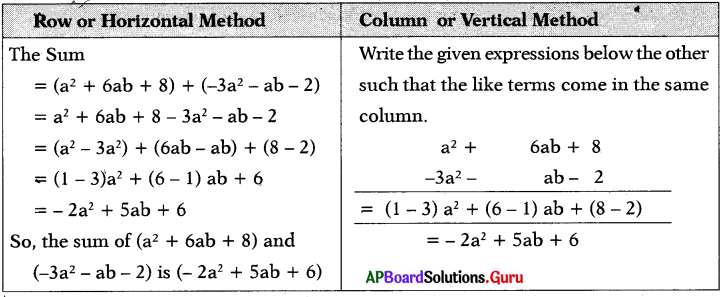(iii) – p2 + 2p – 10; 4 – 5p – 2p2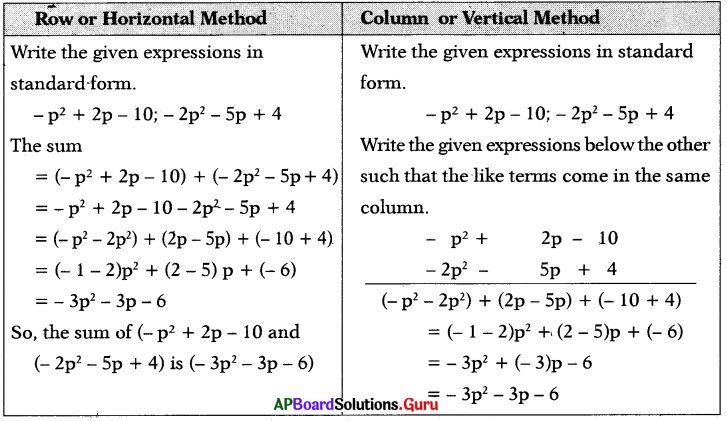Question 4.
Subtract the second expression from the first expression:
(i) 2x + y , x – y
Let A = 2x + y and B = x – y
A – B = A + (- B) Additive inverse of B is
-B = – (x – y) = – x + y
∴ A – B = A + (- B)
= 2x + y + (- x + y)
= 2x + y – x + y
= 2x – x + y + y
= (2 – 1)x + (1 + 1)y
∴ A – B = x + 2y(ii) a + 2b + c, – a – b – 3c
Let A = a + 2b + c and B = – a – b – 3c
A – B = A + (- B)
– B = – (- a – b – 3c)
= a + b + 3c
∴ A – B = A + (- B)
= a + 2b + c + (a + b + 3c)
= a + 2b + c + a + b + 3c
= (a + a) + (2b + lb) + (c + 3c)
∴ A – B = 2a + 3b + 4c

(iii) 2l2 – 3lm + 5m2, 3l2 – 4lm + 6m2
Let A = 2l2 – 3lm + 5m2 and
B = 3l2 – 4lm + 6m2
A – B = A + (- B)
– B = – (3t2 – 4lm + 6m2)
= – 3l2 + 4lm – 6m2
∴ A – B = A + (- B)
= (2l2 – 3lm + 5m2) + (- 3l2 + 4lm – 6m2)
= 2l2 – 3lm + 5m2 – 3l2 + 4lm – 6m2
= 2l2 – 3l2 – 3lm + 4lm + 5m2 – 6m2
= (2 – 3)l2 + (- 3 + 4)lm + (5 – 6)m2
= (- 1) l2 + 1 lm + (- 1)m2
∴ A – B = – l2 + lm – m2

(iv) 7 – x – 3x2, 2x2 – 5x – 3
Let A = 7 – x – 3x2 and B = 2x2 – 5x – 3
Write the given expressions in standard form.
∴ A = – 3x2 – x + 7 and B = 2x2 – 5x – 3
A – B = A + (- B)
– B = – (2x2 – 5x – 3)
= – 2x2 + 5x + 3
∴ A – B = A + (- B)
= (- 3x2 – x + 7) + (- 2x2 + 5x + 3)
= – 3x2 – x + 7 – 2x2 + 5x + 3
= (- 3x2 – 2x2) + (- x + 5x) + (7 + 3)
= (- 3 – 2)x2 + (- 1 + 5)x + 10
∴ A – B = – 5x2 + 4x + 10(v) 6m3 + 4m2 + 7m – 3, 2m3 + 4
Let A = 6m3 + 4m2 + 7m – 3 and
B = 2 m2 + 4
A – B = A + (- B)
– B = – (2m3 + 4)
= – 2m3 – 4
∴ A – B = A + (- B)
= (6m3 + 4m2 + 7m – 3) + (- 2m3 – 4)
= 6m3 + 4m2 + 7m – 3 – 2m3 – 4
= (6m3 – 2m3) + 4m2 + 7m + (- 3 – 4)
= (6 – 2)m3 + 4m2 + 7m + (- 7)
∴ A – B = 4m3 + 4m2 + 7m – 7

Question 5.
Find the perimeter of the beside rect¬angle whose length is 6x + y and breadth is 3x – 2y.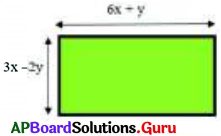Given length of rectangle l = 6x + y
breadth b = 3x – 2y
Perimeter of Rectangle = 2 (l + b)
= 2[(6x + y) + (3x – 2y)]
= 2[6x + y + 3x – 2y]
= 2[(6 + 3)x + (1 – 2)y]
= 2[9x + (- 1) y]
= 2[9x – 1y]
= 2 × (9x) – 2 × (1y)
∴ Perimeter of rectangle = (18x – 2y) units.Question 6.
Find the perimeter of triangle whose sides are a + 3b, a – b and 2a – b.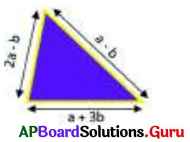Let the sides of triangle are
x = a + 3b, y = a – b and z = 2a – b
Perimeter of triangle = x + y + z
= (a + 3b) + (a – b) + (2a – b)
= a + 3b + a – b + 2a – b
= (a + a + 2a) + (3b – b – b)
= (1 + 1 + 2)a + (3 – 1 – 1)b
Perimeter of triangle.
= (4a + b) units.

Question 7.
Subtract the sum of x2 – 5xy + 2y2 and y2 – 2xy – 3x2 from the sum of 6x2 – 8xy – y2 and 2xy – 2y2 – x2.
Given expressions are
x2 – 5xy + 2y2 and y2 – 2xy – 3x2 and 6x2 – 8xy – y2 and 2xy – 2y2 – x2
Write the given expressions in the standard form
x2 – 5xy + 2y2 and – 3x2 – 2xy + y2 and 6x2 – 8xy – y2 and – x2 + 2xy – 2y2
Let the Sum
A = (x2 – 5xy + 2y2) + (- 3x2 – 2xy + y2)
= x2 – 5xy + 2y2 – 3x2 – 2xy + y2
= x2 – 3x2 – 5xy – 2xy + 2y2 + y2
= (1 – 3)x2 + (- 5 – 2)xy + (2 + 1)y2
A = – 2x2 – 7xy + 3y2

Let the Sum
B = (6x2 – 8xy – y2) + (- x2 + 2xy – 2y2)
= 6x2 – 8xy – y2 – x2 + 2xy – 2y2
= (6 – 1 )x2 + (- 8 + 2)xy + (- 1 – 2)y2
B = 5x2 – 6xy – 3y2
B – A = B + (- A)
– A = – (A)
= – (- 2x2 – 7xy + 3y2)
∴ – A = 2x2 + 7xy – 3y2
B – A = B + (- A)
= (5x2 – 6xy – 3y2) + (2x2 + 7xy – 3y2)
= (5 + 2)x2 + (- 6 + 7)xy + (- 3 – 3)y2
∴ B – A = 7x2 + xy – 6y2Question 8.
What should be added to 1 + 2p – 3P2 to get p2 – p – 1 ?
Given expressions are
1 + 2p – 3p2 and p2 – p – 1
Write the given expressions in the standard form.
– 3p2 + 2p + 1 and p2 – p – 1
Let A should be added to B to get C.
i.e. A + B = C
∴ A = C – B
Let B = – 3p2 + 2p + 1 and
C = p2 – p – 1
A = C + (- B )
– B = – (- 3p2 + 2p + 1) .
– B = 3p2 – 2p – 1 .
A = (p2 – p – 1) + (3p2 – 2p – 1)
= p2 – p – 1 + 3p2 – 2p – 1
= (1 + 3)p2 + (- 1 – 2)p + (- 1 – 1)
∴ A = 4p2 – 3p – 2
∴ 4p2 – 3p – 2 is added to 1 + 2p – 3p2 to get p2 – p – 1.

Question 9.
What should be taken away from 3a2 – 4b2 + 5ab + 20 to get – a2 – b2 + 6ab + 3 ?
Given expressions are
3a2 – 4b2 + 5ab + 20 and – a2 – b2 + 6ab +3
Let A be taken away from B to get C. that is A = B – C = B + (- C)
Let B = 3a2 – 4b2 + 5ab + 20 and C = – a2 – b2 + 6ab + 3 Additive inverse of C is
(- C) = – (- a2 – b2 + 6ab + 3)
= a2 + b2 – 6ab – 3
A = B + (- C)
= (3a2 – 4b2 + 5ab + 20) + (a2 + b2 – 6ab – 3)
= 3a2 – 4b2 + 5ab + 20 + a2 + b2 – 6ab – 3
= 3a2 + a2 – 4b2 + b2 + 5ab – 6ab + 20 – 3
= (3 + 1)a2 + (- 4 + 1)b2 + (5 – 6) ab + (20 – 3)
A = 4a2 – 3b2 – 1 ab + 17
So, 4a2 – 3b2 – 1 ab + 17 is taken away from 3a2 – 4b2 + 5ab + 20 to get – a2 – b2 + 6ab + 3.Question 10.
If A = 4x2 + y2 – 6xy;
B = 3y2 + 12x2 + 8xy;
C = 6x2 + 8y2 + 6xy then,
find(i) A + B + C (ii) (A – B) – C
Given A = 4x2 + y2 – 6xy
B = 3y2 + 12x2 + 8xy
C = 6x2 + 8y2 + 6xy
Write the given expressions in standard form.
A = 4x2 – 6xy + y2
B = 12x2 + 8xy + 3y2
C = 6x2 + 6xy + 8y2

(i) A + B + C = (4x2 – 6xy + y2) + (12x2 + 8xy + 3y2) + (6x2 + 6xy + 8y2)
= 4x2 – 6xy + y2 + 12x2 + 8xy + 3y2 + 6x2 + 6xy + 8y2
= (4x2 + 12x2 + 6x2) + (- 6xy + 8xy + 6xy) + (y2 + 3y2 + 8y2)
= (4 + 12 + 6) x2 + (- 6 + 8 + 6) xy + (1 + 3 + 8)y2
∴ A + B + C = 22x2 + 8xy + 12y2

(ii) (A – B) – C
A + (- B) + (- C)